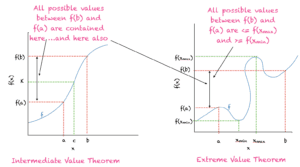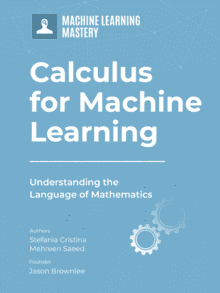# A Gentle Introduction to Continuous Functions

Many areas of calculus require an understanding of continuous functions. The characteristics of continuous functions, and the study of points of discontinuity are of great interest to the mathematical community. Because of their important properties, continuous functions have practical applications in machine learning algorithms and optimization methods.

In this tutorial, you will discover what continuous functions are, their properties, and two important theorems in the study of optimization algorithms, i.e., intermediate value theorem and extreme value theorem.

After completing this tutorial, you will know:

• Definition of continuous functions
• Intermediate value theorem
• Extreme value theorem

Let’s get started.A Gentle Introduction to continuous functions Photo by Jeeni Khala, some rights reserved.

## Tutorial Overview

This tutorial is divided into 2 parts; they are:

1. Definition of continuous functions
1. Informal definition
2. Formal definition
2. Theorems
1. Intermediate value theorem
2. Extreme value theorem

## Prerequisites

This tutorial requires an understanding of the concept of limits. To refresh your memory, you can take a look at limits and continuity, where continuous functions are also briefly defined. In this tutorial we’ll go into more details.

We’ll also make use of intervals. So square brackets mean closed intervals (include the boundary points) and parenthesis mean open intervals (do not include boundary points), for example,

• [a,b] means a<=x<=b
• (a,b) means a<x<b
• [a,b) means a<=x<b

From the above, you can note that an interval can be open on one side and closed on the other.

As a last point, we’ll only be discussing real functions defined over real numbers. We won’t be discussing complex numbers or functions defined on the complex plane.

## An Informal Definition of Continuous Functions

Suppose we have a function f(x). We can easily check if it is continuous between two points a and b, if we can plot the graph of f(x) without lifting our hand. As an example, consider a straight line defined as:

f(x)=2x+1

We can draw the straight line between [0,1] without lifting our hand. In fact, we can draw this line between any two values of x and we won’t have to lift our hand (see figure below). Hence, this function is continuous over the entire domain of real numbers. Now let’s see what happens when we plot the ceil function:

The ceil function has a value of 1 on the interval (0,1], for example, ceil(0.5)= 1, ceil(0.7) = 1, and so on. As a result, the function is continuous over the domain (0,1]. If we adjust the interval to (0,2], ceil(x) jumps to 2 as soon as x>1. To plot ceil(x) for the domain (0,2], we must now lift our hand and start plotting again at x=2. As a result, the ceil function isn’t a continuous function.

If the function is continuous over the entire domain of real numbers, then it is a continuous function as a whole, otherwise, it is not continuous as whole. For the later type of functions, we can check over which interval they are continuous.

## A Formal Definition

A function f(x) is continuous at a point a, if the function’s value approaches f(a) when x approaches a. Hence to test the continuity of a function at a point x=a, check the following:

1. f(a) should exist
2. f(x) has a limit as x approaches a
3. The limit of f(x) as x->a is equal to f(a)

If all of the above hold true, then the function is continuous at the point a.

## Examples

Some examples are listed below and also shown in the figure:

• f(x) = 1/x is not continuous as it is not defined at x=0. However, the function is continuous for the domain x>0.
• All polynomial functions are continuous functions.
• The trigonometric functions sin(x) and cos(x) are continuous and oscillate between the values -1 and 1.
• The trigonometric function tan(x) is not continuous as it is undefined at x=𝜋/2, x=-𝜋/2, etc.
• sqrt(x) is not continuous as it is not defined for x<0.
• |x| is continuous everywhere.

## Connection of Continuity with Function Derivatives

From the definition of continuity in terms of limits, we have an alternative definition. f(x) is continuous at x, if:

f(x+h)-f(x)→ 0 when (h→0)

Let’s look at the definition of a derivative:

f'(x) = lim(h→0) (f(x+h)-f(x))/h

Hence, if f'(x) exists at a point a, then the function is continuous at a. The converse is not always true. A function may be continuous at a point a, but f'(a) may not exist. For example, in the above graph |x| is continuous everywhere. We can draw it without lifting our hand, however, at x=0 its derivative does not exist because of the sharp turn in the curve.

## Intermediate Value Theorem

The intermediate value theorem states that:

If:

• function f(x) is continuous on [a,b]
• and f(a) <= K <= f(b)

then:

• There is a point c between a and b, i.e., a<=c<=b such that f(c) = K

In very easy words, this theorem says that if a function is continuous over [a,b], then all values of the function between f(a) and f(b) will exist within this interval as shown in the figure below.Illustration of intermediate value theorem (left) and extreme value theorem (right)

## Extreme Value Theorem

This theorem states that:

If:

• function f(x) is continuous on [a,b]

then:

• There are points x_min and x_max inside the interval [a,b], i.e.,
• a<=x_min<=b
• a<=x_max<=b
• and the function f(x) has a minimum value f(x_min), and a maximum value f(x_max), i.e.,
• f(x_min)<=f(x)<=f(x_max) when a<=x<=b

In simple words a continuous function always has a minimum and maximum value within an interval as shown in the above figure.

## Continuous Functions and Optimization

Continuous functions are very important in the study of optimization problems. We can see that the extreme value theorem guarantees that within an interval, there will always be a point where the function has a maximum value. The same can be said for a minimum value. Many optimization algorithms are derived from this fundamental property and can perform amazing tasks.

## Extensions

This section lists some ideas for extending the tutorial that you may wish to explore.

• Converging and diverging sequences
• Weierstrass and Jordan definitions of continuous functions based on infinitesimally small constants

If you explore any of these extensions, I’d love to know. Post your findings in the comments below.

This section provides more resources on the topic if you are looking to go deeper.

### Books

• Thomas’ Calculus, 14th edition, 2017. (based on the original works of George B. Thomas, revised by Joel Hass, Christopher Heil, Maurice Weir)
• Calculus, 3rd Edition, 2017. (Gilbert Strang)
• Calculus, 8th edition, 2015. (James Stewart)

## Summary

In this tutorial, you discovered the concept of continuous functions.

Specifically, you learned:

• What are continuous functions
• The formal and informal definitions of continuous functions
• Points of discontinuity
• Intermediate value theorem
• Extreme value theorem
• Why continuous functions are important

## Get a Handle on Calculus for Machine Learning!#### Feel Smarter with Calculus Concepts

...by getting a better sense on the calculus symbols and terms

Discover how in my new Ebook:
Calculus for Machine Learning

It provides self-study tutorials with full working code on:
differntiation, gradient, Lagrangian mutiplier approach, Jacobian matrix, and much more...

### One Response to A Gentle Introduction to Continuous Functions

1.Toby July 27, 2021 at 2:46 am #

Nice article, but the image for the Extreme Value Theorem plot is confusing as it makes it look like f(x) can produce multiple y values – i.e. the line crosses back over itself on the x-axis.